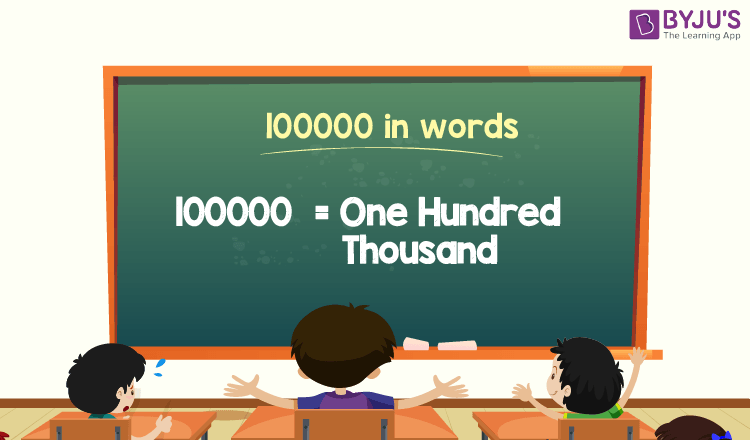# 100000 in Words

100000 in words is One Hundred Thousand. The number name of 100000 is written using the place value of a number, such as ones, tens, hundreds, thousands, etc. For example, if you have saved 100000 in 2 months, then you can write, “I have saved one hundred thousand in two months”.

 100000 in Words: One Hundred Thousand

In this article, we will learn how to write 100000 in words, i.e., the spelling of 100000 in words, and look at many solved examples.

## How to Write 100000 in Words?The place value chart helps to write the number 100000 in words. Using the ones, tens, hundreds, and thousands place value of each digit in the number 100000, we can easily write the number name of 100000.

For a number 100000,

The digit in one’s place = 0

The digit in ten’s place = 0

The digit in the hundred’s place = 0

The digit in the thousand’s place = 100

Thus, 100000 is represented in the place value chart is as follows:

 Thousands Hundreds Tens Ones 100 0 0 0

Therefore, the number 100000 in words is One Hundred Thousand.

### Examples

Example 1:

Determine the value of 70000 + 30000. Express the value in words.

Solution:

Given expression: 70000 + 30000

⇒ 70000 + 30000 = 100000

So, the value of 70000 + 30000 is 100000.

Hence, 100000 in words is one hundred thousand.

Example 2:

What is the value of one hundred twenty-five thousand minus twenty-five thousand in words?

Solution:

One hundred twenty-five thousand = 125000

Twenty-five thousand = 25000

One hundred twenty-five thousand minus twenty-five thousand = 125000 – 25000 = 100000

Hence, the value of one hundred twenty-five thousand minus twenty-five thousand is one hundred thousand in words.

## Frequently Asked Questions on 100000 in Words

Q1

### Write 100000 in words.

100000 in words is one hundred thousand.

Q2

### What is the value of 85000 + 15000? Express the value in words.

Simplifying 85000 + 15000, we get 100000. Hence, 100000 in words is one hundred thousand.

Q3

### How to write one hundred thousand in numbers?

One hundred thousand in numbers is 100000.

Test your Knowledge on 100000 in Words